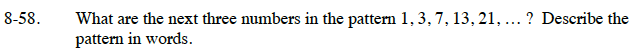### Home > CC1 > Chapter 8 > Lesson 8.1.4 > Problem8-58

8-58.

What are the next three numbers in the pattern: 1, 3, 7, 13, 21, …? Describe the pattern in words. Homework Help ✎Write down the differences between each number and the number after it.
There is a pattern in the amounts by which the numbers increase.

The difference between 1 and 3 is 2, the difference between 3 and 7 is 4, the difference between 7 and 13 is 6, and the difference between 13 and 21 is 8. The amount added follows the pattern 2, 4, 6, 8, and so on.